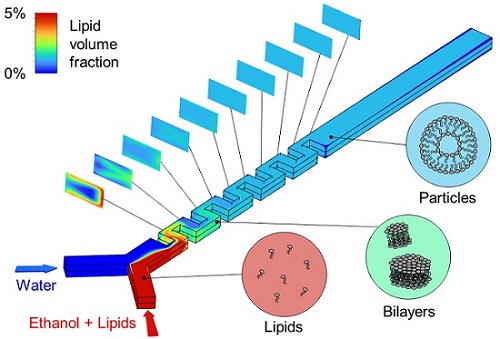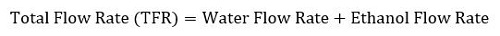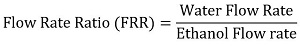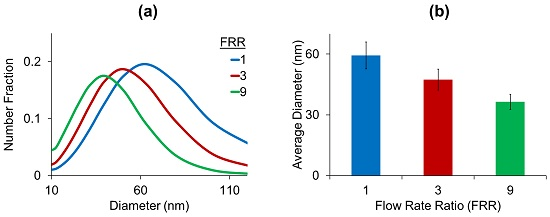# Lipid Nanoparticle Self-assembly for mRNA Vaccine Production

## Technical Challenge

Lipid nanoparticles (LNPs) are used in a variety of pharmaceutical applications including cancer therapies, analgesics, and viral vaccines such as the mRNA vaccines for COVID-19.  The size of LNPs is a critical factor in delivery efficacy: in general, smaller LNPs more easily penetrate tissue and deliver mRNA.

LNP size can be controlled via mixing time: longer mixing times give lipids more time to aggregate, and hence lead to larger aggregates and more heterogeneous size distributions, while shorter mixing times lead to smaller nanoparticles.  However, precise tuning of the LNP size distribution by experimental iteration is time consuming and costly, motivating the need for simulations to complement and expedite experimental development.

## Veryst Solution

Veryst used computational fluid dynamics (CFD) with species transport and the population balance model (PBM) to simulate the simultaneous mixing of ethanol and water and the aggregation of LNPs in a serpentine microfluidic mixer (Figure 1).

This microfluidic device exploits inertial secondary flows to enhance mixing above the diffusion limit.  The ethanol inlet stream contains 50 mM of dissolved lipids. Upon mixing with water, the lipids rapidly precipitate out of solution and self-assemble to form small bilayer fragments.  Subsequently, these fragments aggregate through binary collisions to form successively larger bilayer sheets, eventually closing to form LNPs.  In the Veryst model, binary aggregation is assumed to be the rate-limiting step in LNP self-assembly.Figure 1. Computational fluid dynamics simulation of lipid nanoparticle self-assembly in a serpentine microfluidic mixer.

In industrial pharmaceutical applications, the total flow rate (TFR) of ethanol and water is a critical process parameter for controlling particle size distributions:Figure 2a shows the effect of changing the TFR on the simulated size distribution at the channel outlet. Decreasing the TFR broadens the distribution due to increased particle residence times at lower flow rates, allowing further aggregation to occur and producing larger particles at the outlet. From the simulated size distributions, average LNP diameters were extracted and plotted against the TFR (Figure 2b), showing that the average diameter decreases with TFR.Figure 2. Increasing the total flow rate (TFR) decreases the residence time for lipid bilayer aggregation, which in turn (a) narrows the particle size distribution and (b) decreases the mean particle diameter. In these plots, the flow rate ratio (FRR) is fixed at 3 (three parts water to one part ethanol per unit time).

Another important process parameter is the relative flow rates of water and ethanol, or flow rate ratio (FRR):Figure 3 shows the impact of varying the FRR on the size distribution and average diameter, while keeping the total flow rate fixed.  Figure 3b shows that increasing the FRR tends to decrease the average particle size. This is a direct result of concentration dilution: increasing the FRR, or ratio of water to ethanol, dilutes the overall concentration of lipid aggregates. Since the rate of binary aggregation generally scales with the squared concentration, this dilution tends to slow down aggregation and yield smaller LNPs.Figure 3: Increasing the flow rate ratio (FRR) of water to ethanol dilutes the total lipid concentration and decreases the probability of aggregation, which in turn (a) narrows the particle size distribution and (b) decreases the mean particle diameter. In these plots, the total flow rate (TFR) is fixed at 500 μL/min.

### Conclusion

Veryst predicted the effect of process flow parameters on the size distributions of lipid nanoparticles (LNPs) in a microfluidic chaotic mixer by combining computational fluid dynamics (CFD) with species transport and the population balance model (PBM).

Simulations such as these can help rapidly test and optimize mixer designs and operating protocols for generating LNP populations of a desired size distribution.  Veryst has also simulated other types of mixers, including active laminar mixing and turbulent vortex mixing, as well as scale-up between laminar and turbulent reactive mixers.